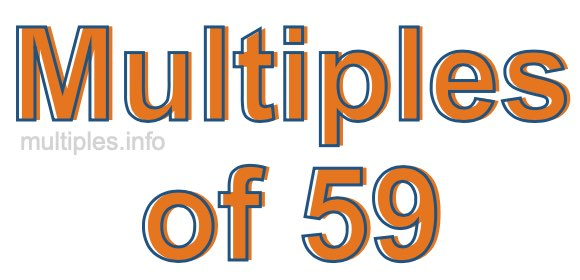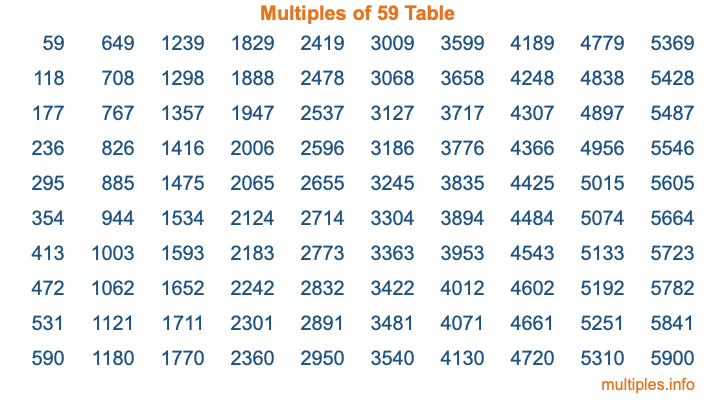Multiples of 59Welcome to the Multiples of 59 page. Here we will first teach you everything you will ever need to know about the multiples of 59, and then give you a study guide summary of everything we taught you to make sure you remember it all. Use this page to look up facts and learn information about the multiples of 59. This page will make you a multiples of fifty-nine expert!

Definition of Multiples of 59
Multiples of 59 are all the numbers that when divided by 59 equal an integer. Each of the multiples of 59 are called a multiple. A multiple of 59 is created by multiplying 59 by an integer.

Therefore, to create a list of multiples of 59, you start with 1 multiplied by 59, then 2 multiplied by 59, then 3 multiplied by 59, and so on for as long as you want. Thus, the list of the first five multiples of 59 is 59, 118, 177, 236, and 295. To see a larger list of multiples of 59, see the printable image of Multiples of 59 further down on this page. We also have a category where you can choose any nth multiple of 59.

Multiples of 59 Checker
The Multiples of 59 Checker below checks to see if any number of your choice is a multiple of 59. In other words, it checks to see if there is any number (integer) that when multiplied by 59 will equal your number. To do that, we divide your number by 59. If the the quotient is an integer, then your number is a multiple of 59.

Is  a multiple of 59?

Least Common Multiple of 59 and ...
A Least Common Multiple (LCM) is the lowest multiple that two or more numbers have in common. This is also called the smallest common multiple or lowest common multiple and is useful to know when you are adding our subtracting fractions. Enter one or more numbers below (59 is already entered) to find the LCM.

Check out our LCM Calculator if you need more details about the Least Common Multiple or if you need the LCM for different numbers for adding and subtraction fractions.

nth Multiple of 59
As we stated above, 59 is the first multiple of 59, 118 is the second multiple of 59, 177 is the third multiple of 59, and so on. Enter a number below to find the nth multiple of 59.

th multiple of 59

Multiples of 59 vs Factors of 59
59 is a multiple of 59 and a factor of 59, but that is where the similarities end. All postive multiples of 59 are 59 or greater than 59. All positive factors of 59 are 59 or less than 59.

Below is the beginning list of multiples of 59 and the factors of 59 so you can compare:

Multiples of 59: 59, 118, 177, 236, 295, etc.

Factors of 59: 1, 59

As you can see, the multiples of 59 are all the numbers that you can divide by 59 to get a whole number. The factors of 59, on the other hand, are all the whole numbers that you can multiply by another whole number to get 59.

It's also interesting to note that if a number (x) is a factor of 59, then 59 will also be a multiple of that number (x).

Multiples of 59 vs Divisors of 59
The divisors of 59 are all the integers that 59 can be divided by evenly. Below is a list of the divisors of 59.

Divisors of 59: 1, 59

The interesting thing to note here is that if you take any multiple of 59 and divide it by a divisor of 59, you will see that the quotient is an integer.

Multiples of 59 Table
Below is an image of the first 100 multiples of 59 in a table. The table is in chronological order, column by column. The first column has the first ten multiples of 59, the second column has the next ten multiples of 59, and so on.The Multiples of 59 Table is also referred to as the 59 Times Table or Times Table of 59. You are welcome to print out our table for your studies.

Negative Multiples of 59
Although not often discussed or needed in math, it is worth mentioning that you can make a list of negative multiples of 59 by multiplying 59 by -1, then by -2, then by -3, and so on, to get the following list of negative multiples of 59:

-59, -118, -177, -236, -295, etc.

Multiples of 59 Summary
Below is a summary of important Multiples of 59 facts that we have discussed on this page. To retain the knowledge on this page, we recommend that you read through the summary and explain to yourself or a study partner why they hold true.

There are an infinite number of multiples of 59.

A multiple of 59 divided by 59 will equal a whole number.

59 divided by a factor of 59 equals a divisor of 59.

The nth multiple of 59 is n times 59.

The largest factor of 59 is equal to the first positive multiple of 59.

59 is a multiple of every factor of 59.

59 is a multiple of 59.

A multiple of 59 divided by a divisor of 59 equals an integer.

59 divided by a divisor of 59 equals a factor of 59.

Any integer times 59 will equal a multiple of 59.

Multiples of a Number
Here you can get the multiples of another number, all with the same attention to detail as we did for multiples of 59 on this page.

Multiples of
Multiples of 60
Did you find our page about multiples of fifty-nine educational? Do you want more knowledge? Check out the multiples of the next number on our list!